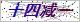网站首页  ◇  产品展示    ◇  仪表备品备件 ◇   搅拌子、搅拌棒 > 226=003=013磁性搅拌棒
••# 磁性搅拌棒

Products

### 产品概述

Z226=003=013型  硅、磷表磁性搅拌棒Z-226=003=013硅、磷表磁性搅拌棒是国外一家公司制造商生产的产品，配套与9210/9211硅磷表。我公司直接从制造商生产厂家大量定期订购，减少中间环节，供货周期现货，价格低廉。欢迎业界好友咨询订购。让您只买对的，不买贵的。

9210硅酸根/磷酸根分析仪备件

接口板                                  09200=A=5000
9210硅表CPU板带显示                      09200=A=1000
9211磷表CPU板带显示                      09200=A=1011

RS485板（MODBUS）                        09125=A=0485/2485
92XX内部总线通讯板                       09200=A=5500
2米内部连接电缆/4米/7米                  370=302=034/304/307
921X测量板（0~1000ppb-SiO2/0~5ppm-PO4）  0921X=A=1500
9210硅表测量板（0~5000ppb-SiO2）         09210=A=1510
9211磷表测量板（0~50ppm-PO4）            09210=A=1520

O-型圈（流速调节螺钉）,4×1mm            35600905040

O-型圈（液位检测器）,38×2mm             35609935308

921X连接板（混合器/加热器）              09210=A=1234
921X混合器                               09210=A=0400

Pt100温度探头                            35900040780

921X光缆                                 09210=A=0500
921X光学镜                               09210=C=0340
921X镜子隔圈                             09210=C=0330
O-型圈（光学镜）,22×1mm                 35609905220

921X2升试剂桶                            495=020=001
921X试剂管沉头                           09210=A=0600
9210硅表化学试剂（不含硫酸）             09210=C=7000
9211磷表化学试剂（0~5ppmPO4,不含硫酸）   09211=C=7000
9211磷表化学试剂（0~50ppmPO4,不含硫酸）  09211=C=7001
9210硅表药剂（一年用量）                 09210=A=0507

### 留言框

• #### 验证码：请输入计算结果（填写阿拉伯数字），如：三加四=7

13865908184